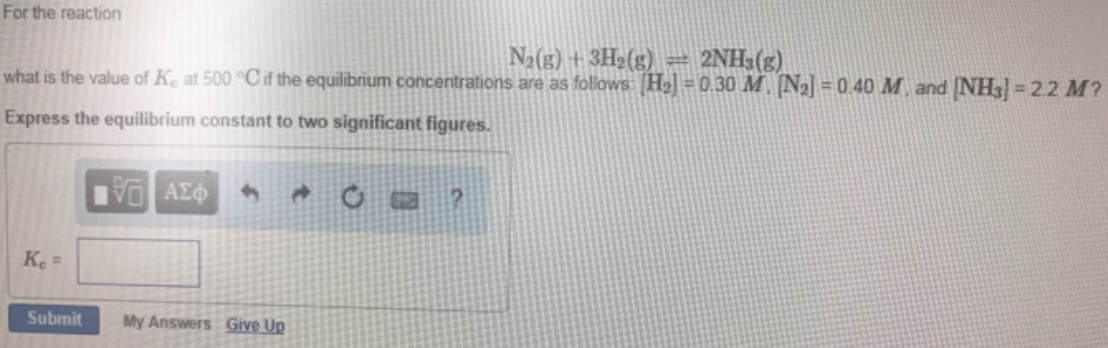# Solution: For the reaction N2 (g) + 3H2 (g) ⇌ 2NH3 (g) what is the value of Kc at 500°C if the equilibrium concentrations are as follows: [H 2] = 0.30 M, [N2] = 0.40 M, and [NH3] = 2.2 M? Express the equilibrium constant to two significant figures.

###### Problem

For the reaction

N2 (g) + 3H2 (g) ⇌ 2NH3 (g)

what is the value of Kc at 500°C if the equilibrium concentrations are as follows: [H 2] = 0.30 M, [N2] = 0.40 M, and [NH3] = 2.2 M?

Express the equilibrium constant to two significant figures.View Complete Written Solution